• +91 9971497814
• info@interviewmaterial.com

# Chapter 7 Triangles Ex-7.4 Interview Questions Answers

### Related Subjects

Question 1 :

Showthat in a right angled triangle, the hypotenuse is the longest side.

Answer 1 :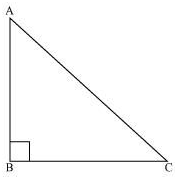Letus consider a right-angled triangle ABC, right-angled at B.

InΔABC,

A + B + C= 180° (Angle sum property of a triangle)

A + 90º + C = 180°

A + C = 90°

Hence,the other two angles have to be acute (i.e., less than 90º).

B is the largest angle inΔABC.

B > Aand B > C

AC > BC and AC > AB

[Inany triangle, the side opposite to the larger (greater) angle is longer.]

Therefore,AC is the largest side in ΔABC.

However,AC is the hypotenuse of ΔABC. Therefore, hypotenuse is the longest side in aright-angled triangle.

Question 2 :

Inthe given figure sides AB and AC of ΔABC are extended to points P and Qrespectively. Also, PBC < QCB. Show that AC > AB.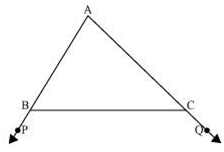Inthe given figure,

ABC + PBC = 180° (Linear pair)

ABC = 180° − PBC… (1)

Also,

ACB + QCB = 180°

ACB = 180° − QCB … (2)

AsPBC < QCB,

180º − PBC > 180º − QCB

ABC > ACB[From equations (1) and (2)]

AC > AB (Side opposite to the largerangle is larger.)

Question 3 :

Inthe given figure, B < A and C< D. Show that AD < BC.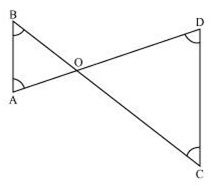InΔAOB,

B < A

AO < BO (Side opposite to smaller angleis smaller) … (1)

InΔCOD,

C < D

OD < OC (Side opposite to smaller angleis smaller) … (2)

Onadding equations (1) and (2), we obtain

AO+ OD < BO + OC

Question 4 :

ABand CD are respectively the smallest and longest sides of a quadrilateral ABCD(see the given figure). Show that A > Cand B > D.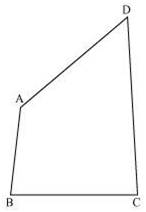Answer 4 :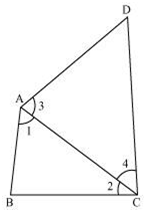Letus join AC.

InΔABC,

AB< BC (AB is the smallest side of quadrilateral ABCD)

2 < 1(Angle opposite to the smaller side is smaller) … (1)

4 < 3(Angle opposite to the smaller side is smaller) … (2)

Onadding equations (1) and (2), we obtain

2 + 4 < 1+ 3

C < A

A > C

Letus join BD.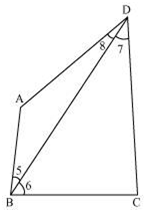InΔABD,

8 < 5(Angle opposite to the smaller side is smaller) … (3)

InΔBDC,

BC< CD (CD is the largest side of quadrilateral ABCD)

7 < 6(Angle opposite to the smaller side is smaller) … (4)

Onadding equations (3) and (4), we obtain

8 + 7 < 5+ 6

D < B

B> D

Question 5 :

Inthe given figure, PR > PQ and PS bisects QPR. Prove that PSR>PSQ.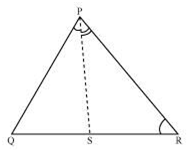AsPR > PQ,

PQR > PRQ(Angle opposite to larger side is larger) … (1)

PSis the bisector of QPR.

∴∠QPS = RPS … (2)

PSR is the exterior angle of ΔPQS.

PSR = PQR+ QPS … (3)

PSQ is the exterior angle of ΔPRS.

PSQ = PRQ+ RPS … (4)

Addingequations (1) and (2), we obtain

PQR + QPS > PRQ+ RPS

PSR > PSQ[Using the values of equations (3) and (4)]

Question 6 :

Showthat of all line segments drawn from a given point not on it, the perpendicularline segment is the shortest.

Answer 6 :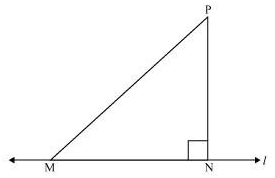Let us take a line l andfrom point P (i.e., not on line l), draw two line segments PN andPM. Let PN be perpendicular to line l and PM is drawn at someother angle.

InΔPNM,

N = 90º

P + N + M= 180º (Angle sum property of a triangle)

P + M = 90º

Clearly,M is an acute angle.

M < N

PN < PM (Side opposite to the smallerangle is smaller)

Similarly, by drawing different linesegments from P to l, it can be proved that PN is smaller incomparison to them.

Therefore,it can be observed that of all line segments drawn from a given point not onit, the perpendicular line segment is the shortest.

Todays Deals### Chapter 7 Triangles Ex-7.4 Contributorskrishan

Name:
Email:

# Latest News# 9000 interview questions in different categories